## Measurement: Volume of Cube and Cuboid - Part 3

Practice Unlimited Questions

#### Units of Volume (l, ml, cm3)

Volume can be measured in several units.

Some of the more common ones are:
• cm3 or cubic centimetres
• millilitres
• litres

Following is the relation between the units of volume:
• 1 litre  =  1000 ml
• 1 ml  =  1 cm3
• 1 litre  =  1000 cm3

#### 2. What is a cubic unit?1 cubic unit is the amount of space that a unit cube occupies.

Or simply,
1 cubic unit = the volume of a unit cube

The object below is made of 6 unit cubes.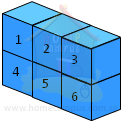Its volume is 6 cubic units.

Here are some other solids that have the same volume (6 cubic units).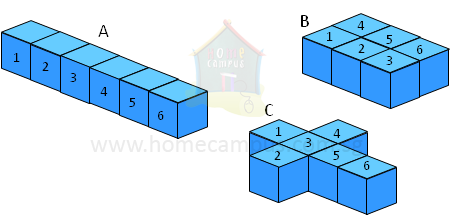#### 3. What is 1 cm3?1 cm3 = the volume of a cube of 1-cm sides

The object below is made of eight 1-cm3 units.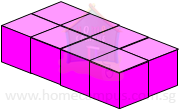Its volume is 8 cm3 or 8 cubic centimetres.

Here are some other solids that have the same volume (8 cm3).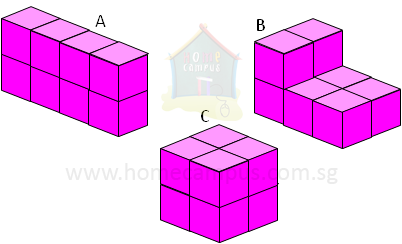#### 4. What is the volume of the cuboid below?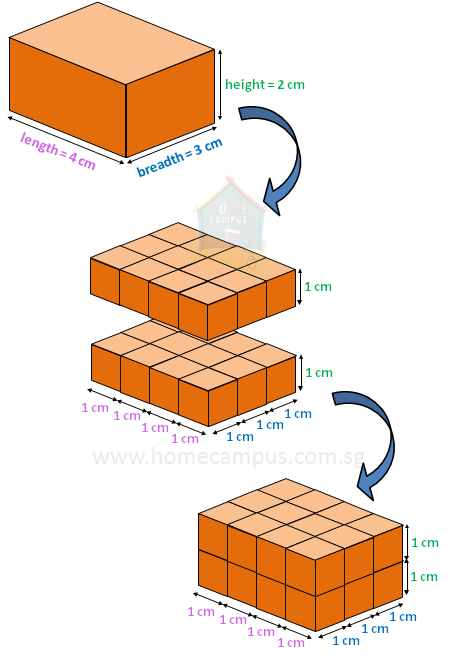Each layer in the cuboid above has 4 × 3 = 12 cubes

Since there are 2 layers in the cuboid, there are
2 × 12 = 24 cubes altogether.

Each of the 24 cubes has a volume of 1 cm3.
So, the volume of the cuboid is 24 × 1 cm3 = 24 cm3.

Volume of a cuboid  =  length  ×  breadth  ×  height

Applying this formula in the example above, we get:

Volume of the given cuboid
= 4 cm × 3 cm × 2 cm
= 24 cm3

#### 5. What is the volume of the cube below?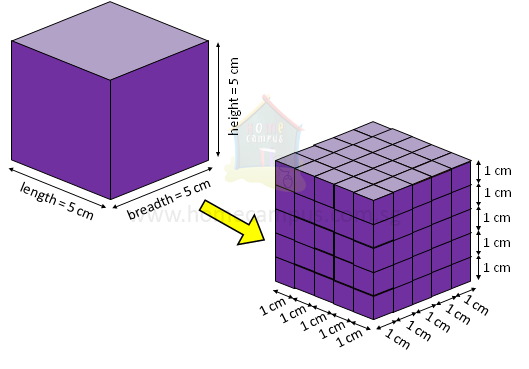A cube is a cuboid with the same length, breadth and height.

Volume of a cube
=  length  ×  breadth  ×  height
=  length  ×  length  ×  length

Volume of the given cube
=  5 cm  ×  5 cm  ×  5 cm
=  125 cm3

#### 6. Calculate the volume of a cuboid-shaped crate that measures 3 m by 2 m by 1.7 m.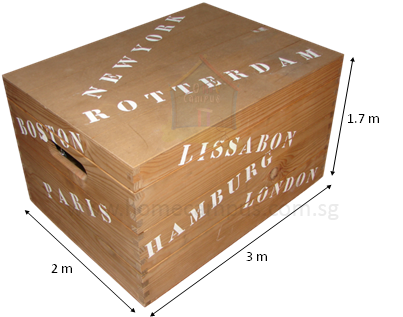Volume of the crate
=  3 m  ×  2 m  ×  1.7 m
=  10.2 m3

The volume of the crate is 10.2 m3.